Test: Simple Stress & Strain Level - 1

# Test: Simple Stress & Strain Level - 1

Test Description

## 20 Questions MCQ Test Engineering Mechanics | Test: Simple Stress & Strain Level - 1

Test: Simple Stress & Strain Level - 1 for Mechanical Engineering 2022 is part of Engineering Mechanics preparation. The Test: Simple Stress & Strain Level - 1 questions and answers have been prepared according to the Mechanical Engineering exam syllabus.The Test: Simple Stress & Strain Level - 1 MCQs are made for Mechanical Engineering 2022 Exam. Find important definitions, questions, notes, meanings, examples, exercises, MCQs and online tests for Test: Simple Stress & Strain Level - 1 below.
Solutions of Test: Simple Stress & Strain Level - 1 questions in English are available as part of our Engineering Mechanics for Mechanical Engineering & Test: Simple Stress & Strain Level - 1 solutions in Hindi for Engineering Mechanics course. Download more important topics, notes, lectures and mock test series for Mechanical Engineering Exam by signing up for free. Attempt Test: Simple Stress & Strain Level - 1 | 20 questions in 25 minutes | Mock test for Mechanical Engineering preparation | Free important questions MCQ to study Engineering Mechanics for Mechanical Engineering Exam | Download free PDF with solutions
 1 Crore+ students have signed up on EduRev. Have you?
Test: Simple Stress & Strain Level - 1 - Question 1

### If a rod expands freely due to heating, it will develop

Detailed Solution for Test: Simple Stress & Strain Level - 1 - Question 1 If a material expands or contract freely due to heating or cooling, then no stress will develop in the material. But if this expansion and contraction is prevented then internal and because of these internal resisting forces thermal stress develops in the material.

Test: Simple Stress & Strain Level - 1 - Question 2

### The ability of a material to deform without breaking is called

Detailed Solution for Test: Simple Stress & Strain Level - 1 - Question 2

The quality of being easily shaped or moulded is called Plasticity. So Plasticity is the ability of the material to deform without breaking.

Test: Simple Stress & Strain Level - 1 - Question 3

### When mild steel is subjected to a tensile load, its fracture will conform to

Test: Simple Stress & Strain Level - 1 - Question 4

What is the relationship between elastic constants E, G and K?

Detailed Solution for Test: Simple Stress & Strain Level - 1 - Question 4 E = 3K(1 − 2μ)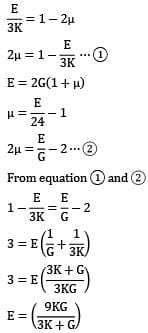From equation 1 and 2Test: Simple Stress & Strain Level - 1 - Question 5

Materials which show direction dependent properties are called

Detailed Solution for Test: Simple Stress & Strain Level - 1 - Question 5 Homogeneous Material: Those materials which have the same properties at all the points.

Viscoelastic Materials:-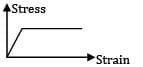Isotropic Materials: Those materials which have the same elastic properties in all the directions.

Anisotropic Materials: Those materials which show direction dependent properties are called anisotropic materials

Test: Simple Stress & Strain Level - 1 - Question 6

The number of elastic constants for a completely anisotropic elastic material is

Detailed Solution for Test: Simple Stress & Strain Level - 1 - Question 6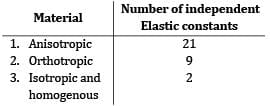Test: Simple Stress & Strain Level - 1 - Question 7

Match List-I (Elastic properties of an isotropic elastic material) with List-II (Nature of strain

produced) and select the correct answer using the codes given below the lists.

List-I

a. Young’s modulus

b. Modulus of rigidity

c. Bulk modulus

d. Poisson’s

List-II

1. Shear strain

2. Normal strain

3. Transverse strain

4. Volumetric strain

Codes: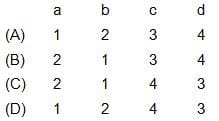Detailed Solution for Test: Simple Stress & Strain Level - 1 - Question 7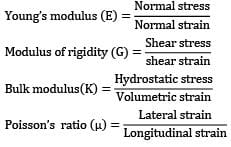Test: Simple Stress & Strain Level - 1 - Question 8

An isotropic elastic material is characterized by

Detailed Solution for Test: Simple Stress & Strain Level - 1 - Question 8 The independent elastic constant will be any two of E, G and μ.

Test: Simple Stress & Strain Level - 1 - Question 9

Shape of true stress-strain curve for a material depends on

Test: Simple Stress & Strain Level - 1 - Question 10

Which one of the following expresses the total elongation of a bar of length L with a constant cross-section of A and modulus of elasticity E hanging vertically and subject to its own weight W?

Detailed Solution for Test: Simple Stress & Strain Level - 1 - Question 10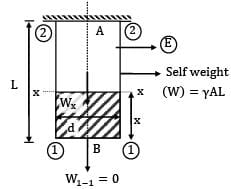Let γ is the weight density.

Wx−x = γAx

at x = 0 ⇒ W1−1 = 0

atx = L ⇒ W2−2 = γAL

Consider a strip at a distance x from section (1) − (1)of dx length

Elongation of the strip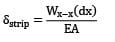Total elongation of bar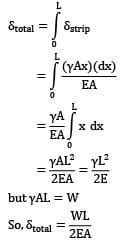Test: Simple Stress & Strain Level - 1 - Question 11

Which one of the following is correct in respect of Poisson’s ratio μ limits for an isotropic elastic solid?

Detailed Solution for Test: Simple Stress & Strain Level - 1 - Question 11 For isotropic elastic solids, μ values can lie between −1 to 0.5 in order to satisfy positive values of E, G and K.

Test: Simple Stress & Strain Level - 1 - Question 12

A bar of copper and steel form a composite system which is heated through a temperature of 40℃. The stress induced in the copper bar is (αcopper > αsteel ).

Detailed Solution for Test: Simple Stress & Strain Level - 1 - Question 12 As thermal coefficient of expansion (α) is more for copper in comparison to steel. So during heating, the tendency of copper is to elongate more, which will be restricted by steel. So copper will experience compressive stress while steel will experience tensile stress.

Test: Simple Stress & Strain Level - 1 - Question 13

The stress-strain curve of an ideal elastic strain hardening material will be as

Test: Simple Stress & Strain Level - 1 - Question 14

A material which undergoes no deformation till its yield point is reached and then it flow at a constant stress is

Test: Simple Stress & Strain Level - 1 - Question 15

In a simple tension test, Hooke’s law is valid upto the

Detailed Solution for Test: Simple Stress & Strain Level - 1 - Question 15

Hooke's law is valid upto the limit of proportionality .because it follows the straight-line equation upto the limit of proportionality. after the limit of proportionality, the elastic limit comes. between the limit of proportionality and elastic limit, the line follows the curve line. normally proportional limit and elastic limit very close to each other that's why we consider elastic limit instead of proportional limit for easy calculation.

Test: Simple Stress & Strain Level - 1 - Question 16

E, G, K and μ represent the elastic modulus, shear modulus, bulk modulus and poisson’s ratio respectively of a linearly elastic, isotropic and homogeneous material. To express the stress-strain relations completely for this material, at least

Detailed Solution for Test: Simple Stress & Strain Level - 1 - Question 16 E = 3k (1 − 2μ) E = 2G (1 + μ) There are two equations and four unknown, so to express the stress-strain relations completely, at least any two of the four must be known. Remaining two can be found out by the equations.

Test: Simple Stress & Strain Level - 1 - Question 17

The Poission’s ratio of a material which has young’s modulus of 120 GPa and shear modulus of 50 GPa, is

Detailed Solution for Test: Simple Stress & Strain Level - 1 - Question 17 E = 2G (1 + μ)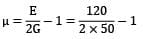μ = 0.2

Test: Simple Stress & Strain Level - 1 - Question 18

A block 100 mm x 100 mm base and 10 mm height. When we apply a tangential force 10 kN to the upper face, it is displaced 1 mm relative to lower face. Then the shear strain in the element is

Detailed Solution for Test: Simple Stress & Strain Level - 1 - Question 18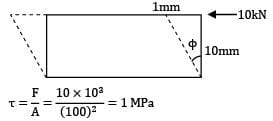tan ϕ = 1 / 10 = 0.1 radian

Question_Type: 5

Test: Simple Stress & Strain Level - 1 - Question 19

A block 100 mm 100 mm base and 10 mm height. When we apply a tangential force 10 kN to the upper edge it is displaced 1 mm relative to lower face.Then the direct shear stress in the element is:

Detailed Solution for Test: Simple Stress & Strain Level - 1 - Question 19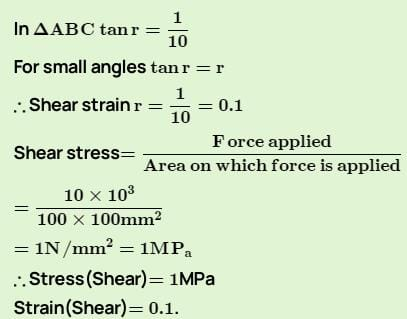Test: Simple Stress & Strain Level - 1 - Question 20

A 10 mm diameter of mild steel of elastic modulus 200 x 109Pa is subjected to a tensile load of 50000N, taking it just beyond its yield point. The elastic recovery of strain that would occur up on removal of tensile load will be

Detailed Solution for Test: Simple Stress & Strain Level - 1 - Question 20 The bar under loading,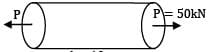d = 10mm

E = 200GPa

Tensile stress in the bar,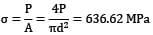Tensile strain will be elastic recovery because bar is loaded up to point of yield point,

∈=σ / E = 3.18 x 10−3

## Engineering Mechanics

17 docs|146 tests
 Use Code STAYHOME200 and get INR 200 additional OFF Use Coupon Code
Information about Test: Simple Stress & Strain Level - 1 Page
In this test you can find the Exam questions for Test: Simple Stress & Strain Level - 1 solved & explained in the simplest way possible. Besides giving Questions and answers for Test: Simple Stress & Strain Level - 1, EduRev gives you an ample number of Online tests for practice

## Engineering Mechanics

17 docs|146 tests